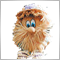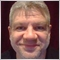555

Hi Alecsey,

I'm testing a trading signal and I read how volume is calculated in my terminal. My question is can I increase the volume on my own? I would like to do it on my own risk, without asking for responsabilities on MQL5 if things go wrong. Could you please tell me how could I do it?

Thanks[Deleted]
landmeister:

Hi Alecsey,

I'm testing a trading signal and I read how volume is calculated in my terminal. My question is can I increase the volume on my own? I would like to do it on my own risk, without asking for responsabilities on MQL5 if things go wrong. Could you please tell me how could I do it?

Thanks

You have 2 ways:

1) Increase your trading balance. After that percentage for volume conversation will be recalculated.

2) Increase % of deposit usage. Tools->Options->Signals "Use no more than x % "  max 95%.555

Aleksey Pak:

You have 2 ways:

1) Increase your trading balance. After that percentage for volume conversation will be recalculated.

2) Increase % of deposit usage. Tools->Options->Signals "Use no more than x % "  max 95%.

My % of deposit usage is at max 95% right now. Is there any other option other than increasing my balance?5

Hi guys,

i've subscribed one signal to my account and metatrade is correctly connected. my question is: the copy work also if the terminal is off?

Thank you.Moderator
270920

AmphybiouS:

Hi guys,

i've subscribed one signal to my account and metatrade is correctly connected. my question is: the copy work also if the terminal is off?

Thank you.

No. The signal is copied while running your local terminal. Or subscribe to virtual hosting - in this case, the local terminal is no longer needed.5

Hello guys,

I'm user of XM micro account, and my pair names at MT4 looks like: EURUSDmicro, GBPUSDmicro, USDJPYmicro.

If I want to follow a signal service, I must do something different?

Or when the signal provider open a EURUSD order my MT4 will open a EURUSDmicro order normally?

Thanks.117

Hello,

what happens if my calculated lot size on a Signal is below Minimum lot size?

for example:

my lot size calculation factor is 6%. What happens when the signal attempts a trade at 0.05 lots? Well 6% of 0.05 lots is 0.003 lots which is below my minimum trade lot size of 0.01 lots.

Is there an error 131 Invalid trade volume and the Signal is not executed on my account?

Or is the Signal automatically rounded up to the minimum lot size of 0.01 and is executed on my account?[Deleted]
Michael Brandl:

Hello,

what happens if my calculated lot size on a Signal is below Minimum lot size?

for example:

my lot size calculation factor is 6%. What happens when the signal attempts a trade at 0.05 lots? Well 6% of 0.05 lots is 0.003 lots which is below my minimum trade lot size of 0.01 lots.

Is there an error 131 Invalid trade volume and the Signal is not executed on my account?

Or is the Signal automatically rounded up to the minimum lot size of 0.01 and is executed on my account?

What rounding scheme is used for Provider's and Subscriber's percentage ratio of deals volume?

The following step-by-step algorithm is used for percentage rounding:

1. If the value is less than 0.01%, it is rounded to 0.001%, i.e. it is assumed to be 0.001%. Examples: 0.007% => 0.001%, 0.000099 => 0.001%.
2. If the value is greater than 0.01% and is less than 0.1%, it is rounded to hundredths. Examples: 0.063% =>0.06%, 0.045 => 0.05%.
3. If the value is greater than 0.1% and is less than 1%, it is rounded to tenths. Examples: 0.11 => 0.1%, 0.25% => 0.3%.
4. If the value is greater than 1% and is less than 10%, it is rounded down to the nearest whole number. Examples: 6.25% => 6%, 7.79% =>7%.
5. If the value is greater than 10% and is less than 100%, it is rounded down to the nearest whole number with step of 5%. Example: 29.7% => 25%.
6. If the value is greater than 100% and is less than 1000%, it is rounded down to the nearest whole number with step of 10%. Example: 129.6% => 120%.

You can see an example of calculations in the General information on Trading Signals for MetaTrader 4 and MetaTrader 5 article.42596

Aleksey Pak:
What rounding scheme is used for Provider's and Subscriber's percentage ratio of deals volume?

The following step-by-step algorithm is used for percentage rounding:

1. If the value is less than 0.01%, it is rounded to 0.001%, i.e. it is assumed to be 0.001%. Examples: 0.007% => 0.001%, 0.000099 => 0.001%.
2. If the value is greater than 0.01% and is less than 0.1%, it is rounded to hundredths. Examples: 0.063% =>0.06%, 0.045 => 0.05%.
3. If the value is greater than 0.1% and is less than 1%, it is rounded to tenths. Examples: 0.11 => 0.1%, 0.25% => 0.3%.
4. If the value is greater than 1% and is less than 10%, it is rounded down to the nearest whole number. Examples: 6.25% => 6%, 7.79% =>7%.
5. If the value is greater than 10% and is less than 100%, it is rounded down to the nearest whole number with step of 5%. Example: 29.7% => 25%.
6. If the value is greater than 100% and is less than 1000%, it is rounded down to the nearest whole number with step of 10%. Example: 129.6% => 120%.

You can see an example of calculations in the General information on Trading Signals for MetaTrader 4 and MetaTrader 5 article.42596

Michael Brandl:

Hello,

what happens if my calculated lot size on a Signal is below Minimum lot size?

for example:

my lot size calculation factor is 6%. What happens when the signal attempts a trade at 0.05 lots? Well 6% of 0.05 lots is 0.003 lots which is below my minimum trade lot size of 0.01 lots.

Is there an error 131 Invalid trade volume and the Signal is not executed on my account?

Or is the Signal automatically rounded up to the minimum lot size of 0.01 and is executed on my account?